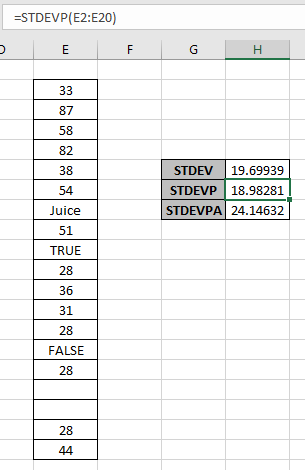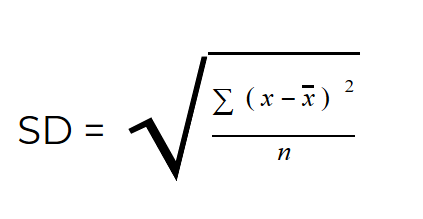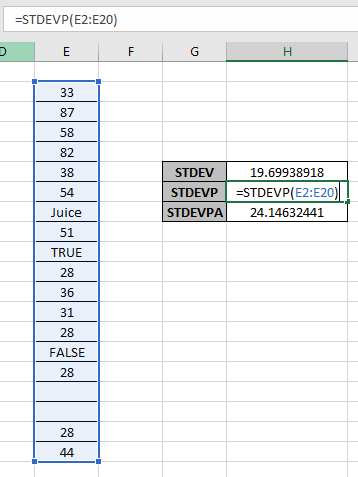# How to use the STDEVP Function in ExcelIn this article, we will learn about how to use the STDEVP function in Excel to calculate the standard deviation  for the range of values.

What is Standard deviation? And What type of Excel functions use it?
STDEVP function is a built-in function to calculate the standard deviation of the range of population data provided. The standard deviation for the set of values is calculated using the formula shown below.Here

1. x is the mean or average of the data.
2. n is the number of values in the data.
3. And x is the different data values.

There are newer and updated versions of STDEV function used for a different set of dataset values.

1. STDEVP : This function is used for dataset having population.
2. STDEV.S : This function used for data set have sample values
3. STDEV.P : This function is a newer version for STDEVP. Excel recommends this over STDEVP
4. STDEVA : This function accepts the text or cell reference values which other STDEVA functions ignore.
5. STDEVPA : This function accepts population as dataset and text and logic_values are considered in the dataset.

STDEVP function in excel returns a number which is the standard deviation, considering population data ignoring text or logical values.

Syntax of STDEVP function:

=STDEVP ( Value 1, [value 2], ...)

value 1 : First value ( necessary )

value 2 : Second value ( optional ). Upto 255 values can be added.

Example:

All of these might be confusing to understand. So, let's test this formula via running it on the example shown below. Here we have some population dataset to test the STDEVP function in Excel.

Here we have a range of values from E2:E20 cell and we need to get the standard deviation for the same using the below formula

Use the formula:

=STDEVP(E2:E20)Here array argument to the function is given using the cell reference method. Using the above formula in the cell to get the standard deviation of the values.The Standard deviation for the population dataset not considering text values and Logic values is 18.98281 whereas STDEV function returns 19.69939 for the sample population. The STDEVPA function considers text and logical values and returns 24.14632. This difference occurs as the value of n in the formula changes, as in STDEVPA case value of n is 19 ( includes text & logic values )  but for STDEV & STDEVP case value of n is 14 ( ignores text & Logic values ).

Here are some observational notes shown below.
Notes:

1. The function is used for the sample population data.
2. If data represents a sample data, use the STDEVA function. STDEVA function is used to calculate the standard deviation for the sample data considering text and logical values.
3. Arguments which are error values, returns error.
4. The function returns the #DIV/0! (division) error, if there’s only text or logic values.

Hope this article about How to use the STDEVP function in Excel is explanatory. Find more articles on statistical function formulas here. If you liked our blogs, share it with your fristarts on Facebook. And also you can follow us on Twitter and Facebook. We would love to hear from you, do let us know how we can improve, complement or innovate our work and make it better for you. Write to us at info@exceltip.com.

Related Articles

How to Use STDEV Function in Excel : Calculate the standard deviation for the data numbers in excel using the STDEV function.

How to use the STDEV.P function in Excel : Calculate the standard deviation  for the population data numbers in excel using the VAR.P function

How to use the DSTDEVP function in Excel : Calculate the standard deviation for the sample data numbers having multiple criteria in excel using the DSTDEVP function

How to use the VAR function in Excel : Calculate the variance for the sample data numbers in excel using the VAR function.

Regressions Analysis in Excel : Regression is an Analysis Tool, which we use for analyzing large amounts of data and making forecasts and predictions in Microsoft Excel.

How to Create Standard Deviation Graph : The standard deviation tells how much the data is clustered around the mean of the data.

Popular Articles:

50 Excel Shortcuts to Increase Your Productivity | Get faster at your task. These 50 shortcuts will make you work even faster on Excel.

The VLOOKUP Function in Excel | This is one of the most used and popular functions of excel that is used to lookup value from different ranges and sheets.

COUNTIF in Excel 2016 | Count values with conditions using this amazing function. You don't need to filter your data to count specific values. Countif function is essential to prepare your dashboard.

How to Use SUMIF Function in Excel | This is another dashboard essential function. This helps you sum up values on specific conditions.

Terms and Conditions of use

The applications/code on this site are distributed as is and without warranties or liability. In no event shall the owner of the copyrights, or the authors of the applications/code be liable for any loss of profit, any problems or any damage resulting from the use or evaluation of the applications/code.